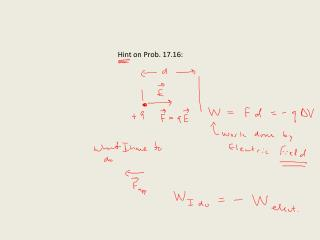DownloadDownload PresentationHint on Prob. 17.16:

Hint on Prob. 17.16:

Télécharger la présentationHint on Prob. 17.16:

- - - - - - - - - - - - - - - - - - - - - - - - - - - E N D - - - - - - - - - - - - - - - - - - - - - - - - - - -
Presentation Transcript

1. Hint on Prob. 17.16:

2. Equipotential and field lines:

3. Equipotential lines field lines:

4. Equipotential lines and the Electric field: Quantitative relation

5. Equipotential lines and the Electric field: Quantitative relation ?

6. Equipotential lines and the Electric field: Quantitative relation

7. Small enough scale, looks like parallel plate field:

8. Formally: Direction: downhill

9. Pretending that V(x) is like a topographic map: The Electric field always points in the direction of steepest descent! B) The magnitude of the electric field is given by the slope in that direction! alternate units for E: V/m

10. Note: color Scheme is backward

11. Example 17.22 where’s V = 0? 5 cm Two possibilities!

12. Example 17.22 where’s V = 0? Possibility #1 5 cm x

13. Example 17.22 where’s V = 0? Possibility #2 x

14. Conductors!

15. Electric fields just outside of a conductor are strong where the conductor is pointy: Dielectric strength of a material = maximum electric field the Material can stand before breaking down. Dielectric strength of air = 3 × 106 V/m Example: Voltage of a 8 cm spark!

16. Capacitance:

17. Capacitance: Must be at different potentials!

18. Capacitance:

19. Capacitance:

20. 10 cm Parallel-plate capacitor: 10 cm 0.1 cm Square plates

21. Parallel-plate capacitor: Q = ?

22. Parallel-plate capacitor: Dielectric constant! Quartz: k = 4.3 Dielectric! 10 cm 0.1 cm Square plates

23. V de G demo again, big and little spheres – spark size Leyden-jar demo